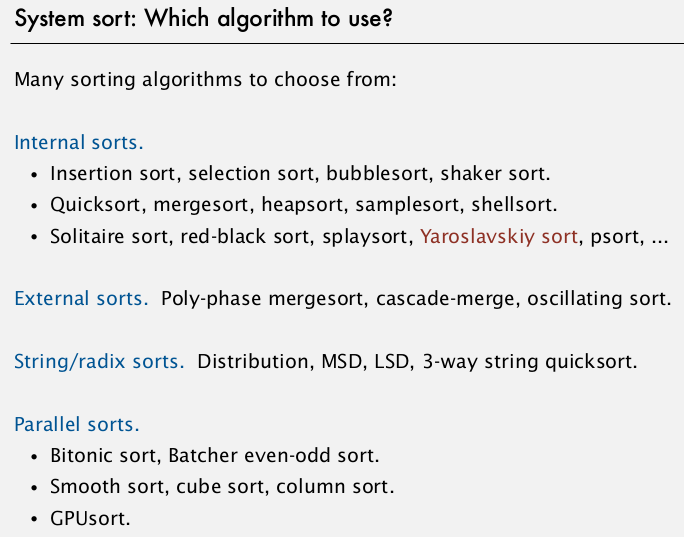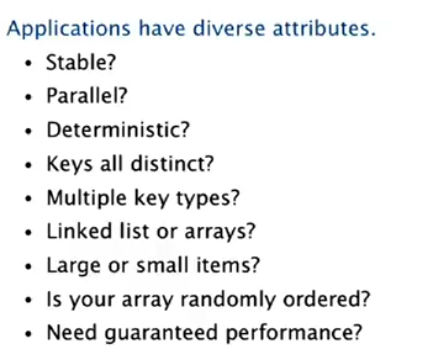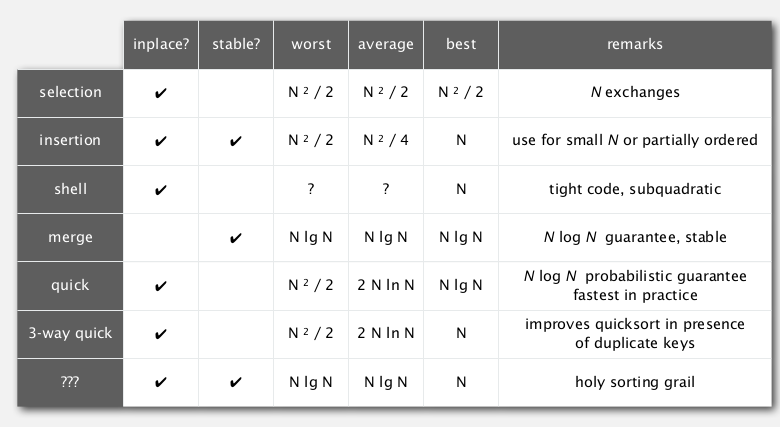# [Algorithms I] Week 3-2 Quicksort

(maybe best algorithm for sorting.)

# 1. Quicksort

Idea:

1. shuffle the array
2. Partition the array into two subarrays to left and right of pivot (*now pivot is *in its final position)

no larger entry to the left of pivot
no smaller entry to the right of pivot

1. sort each subarray recursively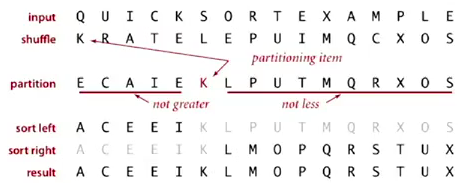## Implemetation

The partition process:

Use 2 pointers i and j (个人觉得用hi, lo, pivot更好...) :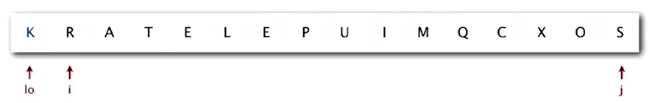→ a[i]>=a[lo], a[j]<=a[lo] (注意是大于等于/小于等于)
⇒ exchange i and j
→ Scan until i and j cross (ie. j<=i)
⇒ finally exchange lo with j

```private static int partition(Comparable[] a, int lo, int hi){
int i=lo+1, j=hi;
while(i<j){
while( i<=hi && less(a[i],a[lo]) ) i++; //a[i]>=a[lo]
while( j>=lo && less(a[lo],a[j]) ) j--; //a[j]<=a[lo]
if(i<j) exch(a,i++,j--);
}
exch(a,lo,j); //exchange pivot with j
return j; //j in its final position
}
```

• test for cross pointers is not trival (ex. edge case: the pivot is the smallest/largest entry in the range)
• i<=hi is necessary !
• for keys equal to a[lo]: better to stop at them

invariance: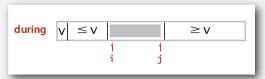Quicksort:

```private static void sort(Comparable[] a, int lo, int hi){
if(hi<=lo) return;
int pivot = partition(a, lo, hi);
sort(a,lo,pivot-1);
sort(a,pivot+1,hi);
return;
}
public static void sort(Comparable[] a){
StdRandom.shuffle(a); // don't forget to shuffle the array
sort(a,0,a.length-1);
}
```

The randomness is preserved: the subarrays after partitionning is still randomly ordered.

## Analysis

Performance: ~40% faster than mergesort.

Best case

# compares = NlgN

(each partition will divide the array in half)

Worst case

# compares = 1/2*N^2

N+(N-1)+...+1
if the array is already in order, each partition will have one subarray of length=0

Average case

proposition
On average, for array with N distinct keys, the #compares = ~2NlnN, #exchanges = ~1/2NlnN.
Proof.*
C(N) := # compares for N entries
pivot 在N个数离的排名是uniform的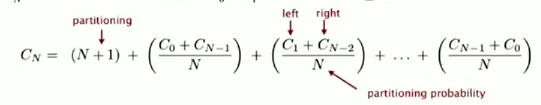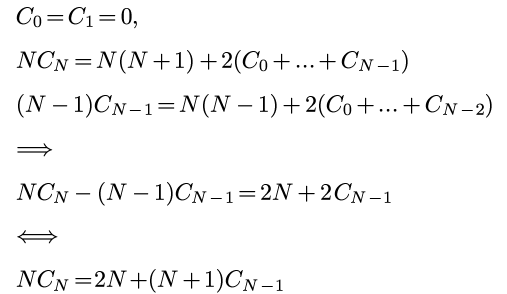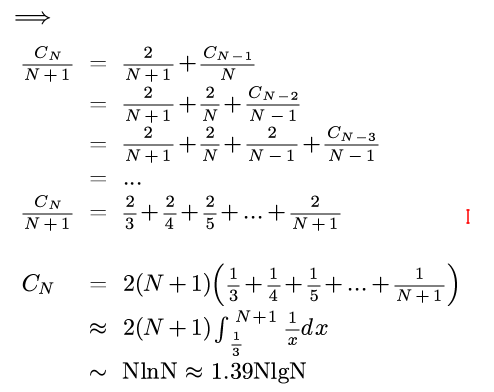(上面最后一行写错了... 是2NlnN...orz) random shuffle: probalistic guarantee against worst case.

Pitfalls
implementations will get quadratic performance if array:

• is sorted or reverse sorted
• has many duplicates (even if randomized)

Staility
Quicksort is NOT stable.
partitionning can make long range exchanges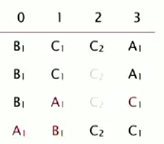## Practical improvements

• cutoff to insertion sort for <10 items

→ ~20% improvement
Or we can leave the small subarrays unsorted and sort them at last using insertion sort

• estimate median by sampling 3 items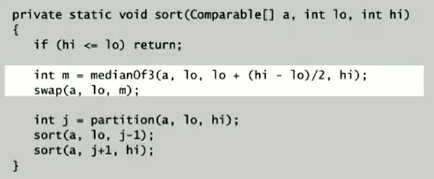→ 10% improvement

# 2. Selection

Goal: given un array, find the kth largest item.

• Upper bound for this problem: NlgN (just sort the array)
• for small k (ex k=1,2,3), the upper bound is N (one-pass/two-pass)
• Lower bound is N: at least have to look at everything

## Quick select

Algo proposed also by Hoare:

1. partition the array into two arrays left of pivot and right of pivot.
2. if pivot==k: return
3. continue the partition for just one of the subarrays

## Implementation

```privater static int partition(Comparable[] a, int lo, int hi){...}

public static Comparable select(Comparable[] a, int k){
StdRandom.shuffle(a);
int lo=0,hi=a.length-1;
while(true){
int j = partition(a,lo,hi);
if(j<k) lo=j+1;
if(j==k) return a[j];
else hi=j-1;
if(hi<=lo) break;
}
return a[k];//这里不太理解为什么会在hi<lo的时候直接返回a[k]
}
```

## Analysis

Proposition
Quick selection takes linear time on average.
*proof *
intuitively, each partition will ct the subarray size in half:
N+N/2+N/4+... = 2N
formal analysis 略...

worst case: quadratic (but very rare to happen)

Theoretical results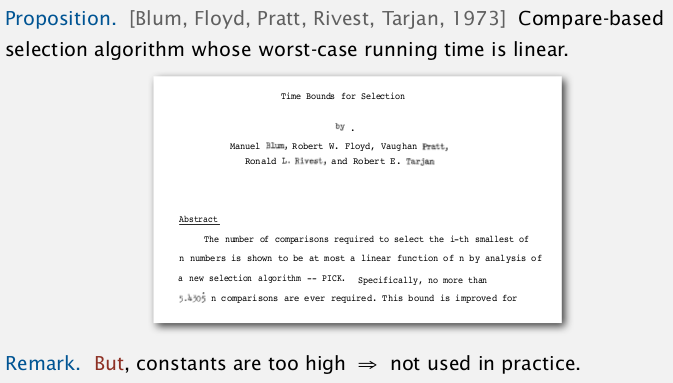# 3. Duplicate keys

if array contains many duplicate keys.

• huge array
• small number of distinct keys

for mergesort
insensitive... always ~NlgN compares.

for quicksort
Will get quadratic time if not stop on equal keys. (found in 1990s)
mistake: put all items equal to pivot *to just one side *
→ N^2 compares if all keys are equal from lo to hi.
correct: put all items equal to pivot in their final place.## 3-way partitionning

(by Dijkstra)
partition the array into 3 parts: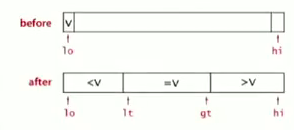Dijkstra's 3-way partition algo:

• lt=lo, gt=hi, i=lo
• if a[i]==v : i++
• if a[i]<v: exch(i,lt), i++, lt++
• if a[i]>v: exch(i,gt), gt--
• repeat until i and gt cross (i>gt)

invariance:

• `[lo, lt)` all < v
• `[lt, i)` all == v
• `(gt, hi]` all >v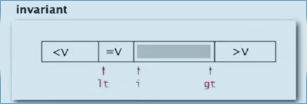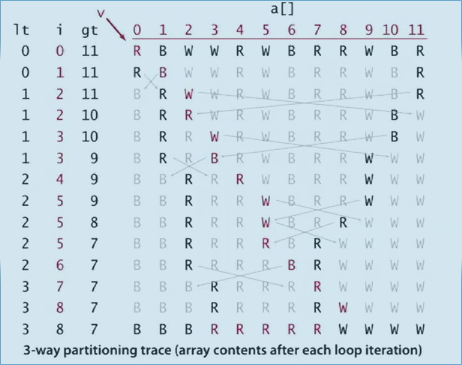## Implementation: 3-way quick sort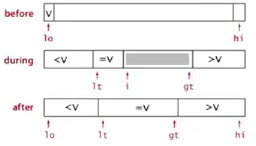```private static void sort(Comparable[] a, int lo, int hi){
if(hi<=lo) return; // 递归函数别忘了先写终止条件...
int lt=lo, gt = hi;
Comparable v = a[lo];
for(int i=lo;i<=gt;){ //不能写 i++
if( less(a[i],v) )
exch(a, i++, lt++);
else if ( less(v,a[i]) )
exch(a,i,gt--);
else // v==a[i]
i++;
}
sort(a, lo, lt-1);
sort(a, gt+1, hi);
}
```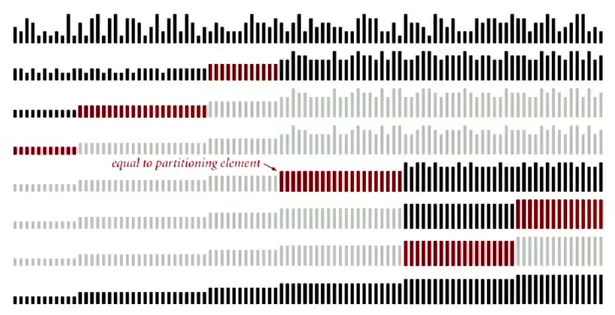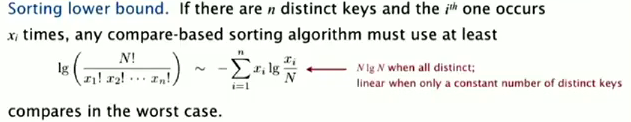And Sedgewick proved that the 3-wy partition is propotional to the lower bound....# 4. System Sorts

Arrays.sort() in java:

`import java.util.Arrays;`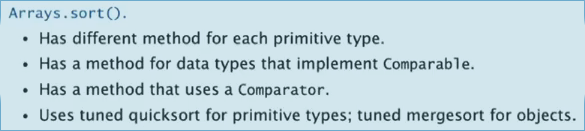quicksort for primitive arrays, mergesort for objects: java设计者认为如果用obj array表示空间不是问题...

Pb in java's system sort: killer input exsit (havn't shuffle)...